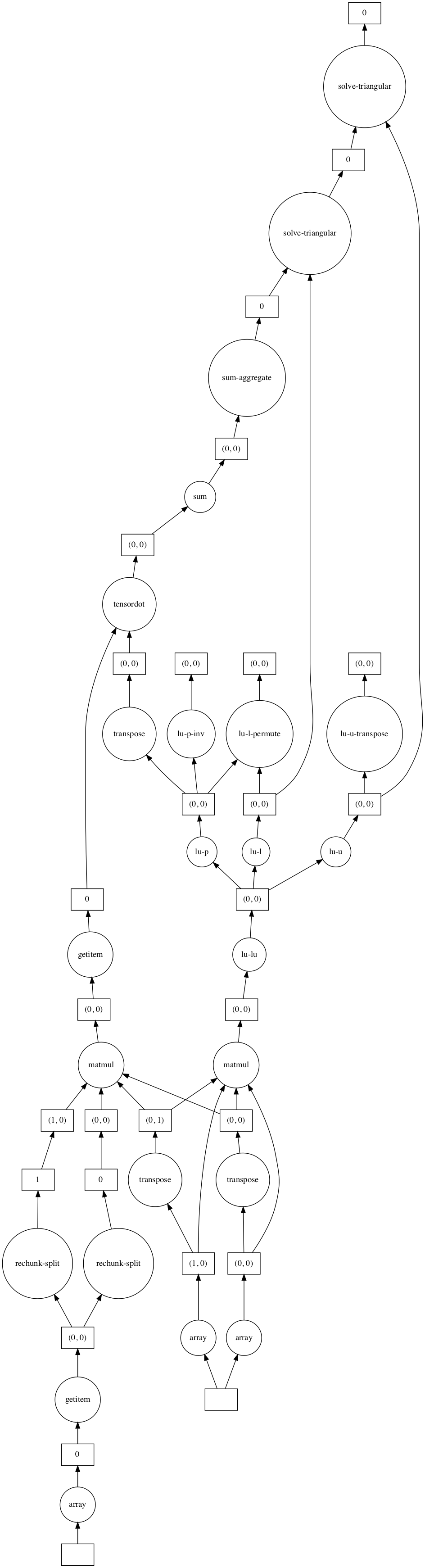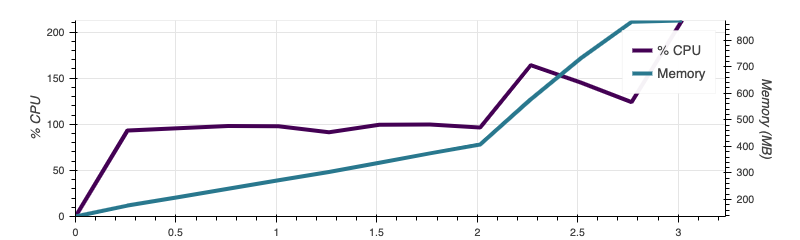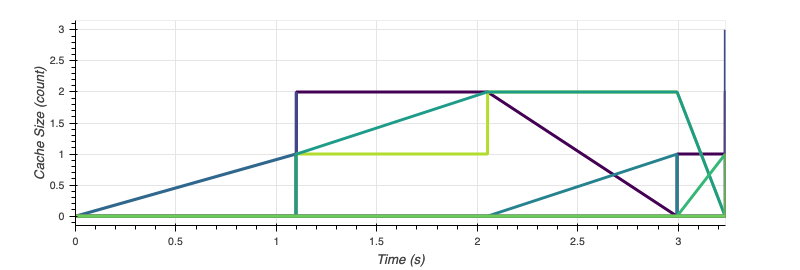# Larger-than-memory regression with PyTables and dask

I have been trying to fit a linear regression and perform some matrix computations using a large dataset which will not fit in memory.

The raw data (about 9 gigabytes) are stored in csv file. I would like to create a regression design matrix using patsy) and then perform matrix computations (e.g. an SVD) and fit the regression model using that design matrix.

Here is my current solution: read the csv file in chunks using pandas; generate (with patsy) and save (with PyTables) the design matrix for each chunk; perform computations with dask.

For demonstration, I’ll generate synthetic data.

``````import numpy as np
import pandas as pd
import tables
from dask import array as da
from dask.diagnostics import Profiler, ResourceProfiler, CacheProfiler, visualize
from patsy import dmatrix
from patsy.contrasts import Treatment

# Generates synthetic covariates
#     chunksize: size of generated chunk
#     measvars: names of quantitative variables
#     catvars: names of categorical variables
#     ncat: number of categories in all categorical variables
def newchunk(chunksize, measvars, catvars, ncat):
d = pd.DataFrame(np.random.randn(chunksize, len(measvars)),
columns = measvars)
for var in catvars:
d[var] = np.random.randint(0, ncat, chunksize)
return d

``````

To start, we choose a reasonable chunk size based on memory limitations.

``````chunksize = 50000 # number of rows per chunk
``````

The following variables are used for generating synthetic data.

``````nchunks = 50 # how many synthetic chunks to generate
measvars = list('abcd') # four quantitative variables
catvars = ['cat1'] # a single categorical variable
ncat = 5
lnames = np.arange(ncat) # the category names for each categorical variable
``````

Create the patsy formula, and form the design matrix for a small amount of data so we know how many columns are in the design matrix:

``````formula = "0 + a + b + c + d + d:c + a:b + a:c + C(cat1, Treatment, levels = lnames)"
pdim = dmatrix(formula, newchunk(1, measvars,  catvars, ncat)).shape
``````

We need a vector of regression coefficients to generate the synthetic response variable.

``````beta = np.arange(0, 1, 1.0 / pdim) # true regression coefficients for generating synthetic data
print(beta)
``````
``````[0.         0.08333333 0.16666667 0.25       0.33333333 0.41666667
0.5        0.58333333 0.66666667 0.75       0.83333333 0.91666667]
``````

We will store the generated design matrix in a compressed HDF5 file. We start by creating the HDF5 file and two PyTables EArrays, which can be enlarged iteratively. We use the EArrays to store the design matrix and the response vector chunk-by-chunk.

``````h5fname = 'design-test.h5'
h5file = tables.open_file(h5fname, mode='w')
fatom = tables.Float64Atom() # the type of data to be stored in the EArrays
Xarray = h5file.create_earray(h5file.root, 'X', fatom,
shape = (0, pdim), # the first dimension will be expanded
filters = tables.Filters(complevel=1,
complib='zlib'),
expectedrows = nchunks * chunksize)
Yarray = h5file.create_earray(h5file.root, 'Y', fatom,
shape = (0,),
filters = tables.Filters(complevel=1,
complib='zlib'),
expectedrows = nchunks * chunksize)

``````

The following loop accomplishes our main task. We loop through each memory-friendly chunk and append the chunk-specific design matrix and response vector to our PyTables arrays.

``````## with a csv file the loop would look like this:
#      for chunk in pd.read_csv(filename, chunksize = chunksize)
for i in range(nchunks):
chunk = newchunk(chunksize, measvars, catvars, ncat) # 'read' a chunk of raw data
xmat = dmatrix(formula, chunk) # design matrix for current chunk
Yarray.append(xmat.dot(beta) + np.random.randn(chunksize)) # synthetic response variable
Xarray.append(xmat)

h5file.close()
``````

We can manipulate the stored arrays using dask arrays.

``````h5read = tables.open_file('design-test.h5', mode='r')
``````

Dask will split these arrays into chunks and perform computations on each chunk. The`visualize` method will display the dask task graph without executing any computations.

``````print((Xmat.shape, Y.shape))
Xmat.visualize()
``````
``````((2500000, 12), (2500000,))
``````We compute the linear regression coefficients by solving a system of equations with dask’s linear algebra API:

``````# fit the regression
betahat = da.linalg.solve(da.matmul(Xmat.T, Xmat), da.matmul(Xmat.T, Y))
``````

The task graph for solving this linear system:

``````betahat.visualize()
``````Once we call `.compute()` on `betahat`, dask executes the task graph and loads the result into memory.

``````with Profiler() as prof, ResourceProfiler(dt=0.25) as rprof, CacheProfiler() as cprof:
betahat_mem = betahat.compute()
``````
``````print(np.round(np.array([betahat_mem, beta]),3).reshape(pdim,2))
``````
``````[[0.    0.082]
[0.166 0.252]
[0.334 0.417]
[0.499 0.583]
[0.667 0.75 ]
[0.833 0.916]
[0.    0.083]
[0.167 0.25 ]
[0.333 0.417]
[0.5   0.583]
[0.667 0.75 ]
[0.833 0.917]]
``````

Dask also provides some profiling tools.

``````visualize([prof, rprof, cprof])
``````